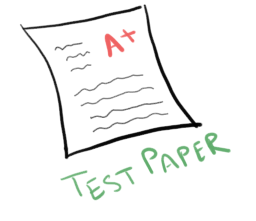# Class 9 Science Gravitation test questionsQuestion 1.
Two particles A and B of mass $m_1$ and $m_2$ respectively are placed at some distance. If the mass of each of the two particles is doubled, keeping the distance between them unchanged, the value of gravitational force between them will be
a. 4 times
b. 2 times
c. 1/2 times
d. 1/4 times

Question 2.
A boy is whirling a stone tied with a string in an horizontal circular path. If the string breaks, the stone
(a) will continue to move in the circular path
(b) will move along a straight line towards the centre of the circular path
(c) will move along a straight line tangential to the circular path
(d) will move along a straight line perpendicular to the circular path away from the boy

Question 3.
An object weighs 20 N when measured on the surface of the earth. What would be its weight when measured on the surface of the moon?

Question 4.
Earth Gravitational pull causes an acceleration $7 m/s^2$ in 2 kg of body somewhere in the space. How much will be the acceleration of 10 Kg mass due to earth gravitational pull at the same place

Question 5.
Why are railway tracks laid on large sized concrete sleepers?

Question 6.
While drawing water from a well, the bucket of water appears to be heavier as it comes out of the water?

Question 7.
Why does a block of plastic releases underwater come up to the surface of water?

Question 8.
A child sitting along a swimming pool feels lighter while lowering his legs in water. Why?

Question 9
A 100 cm3 block has a mass of 395g. Find its relative density
given density of water is 1000 kg/m3

Question 10
On the earth, a stone is thrown from a height in a direction parallel to the earth’s surface while another stone is simultaneously dropped from the same height. Which stone would reach the ground first and why?

Question 11
Differentiate between density and relative density

Question 12
Define
a.Buoyancy
b.Factor of buoyant force
c.Archimedes Principle and its use

Question 13
Explain why
a. Pressure on ground more when man is walking than he is standing?
b. A bucket of water is lighter when in water than when it is taken out of water?
c. An iron nail floats on mercury but sink in water
d. It is easy to walk on sand with flat shoes than with high heel shoes?

Question 14
A iron cube of side 10 cm is kept on a horizontal table. If the density of iron is 8000 kg/m3.Find the pressure on the portion of the table where cube is kept
Take g=10m/s2

Question 15
The dimension of wooden block is $2m \times .25 m \times .10 m$. If relative density of wood is 0.6 calculate the mass of the block in kg
given density of water is 1000 kg/m3

Question 16
A block of wood is kept on the table top. The mass of wooden block is 5 kg and its dimension are $40 \; cm \times 20 \; cm \times 10 \; cm$. Find the pressure exerted by the wooden block on the table if it made it lie  on the table top with its sides of dimension
a. $20 \; cm \times 10 \; cm$
b. $40 \; cm \times 20 \; cm$
Take g=9.8 m/s2

Question 17.
(i)Define thrust?
(ii) Name the factors on which the pressure depends. If a man standing on a sand surface lies down on the sand, how will the pressure exerted on sand by the man be affected in two positions?

Question 18.
What do you understand by 1 Pascal and 1 Newton?

Question 19
Obtain a relation between the weight of an object on the surface of earth and that on moon.
Question 20
On the earth, a stone is thrown from a height in a direction parallel to the earth’s surface while another stone is simultaneously dropped from the same height. Which stone would reach the ground first and why?
Question 21
Prove that if a body is thrown vertically upward, the time of ascent is equal to the time of descent.